# Data Scientists Interview Questions Data Science

If you want to get through the toughest of interviews, start practising the interview questions for Data Science listed here. These are compiled by experts that will help you prepare for your upcoming interviews. Your next interview will surely convert into a job offer if you practice these Data Science interview questions and also help you understand the difference between supervised and unsupervised learning, what is Bias-Variance tradeoff, Selection Bias, and why data cleansing is important in data analysis. Prepare in advance and land your dream career as a Data Scientists, Data Analyst or Data Engineer.

• 4.6 Rating
• 25 Question(s)

## Beginner

Supervised learning is the learning of the model where with input variable ( say, x) and an output variable (say, Y) and an algorithm to map the input to the output. It works using labelled data.

That is, Y = f(X) .

For example, from a basket filled with fruits, you have to arrange the same type of fruits in one place. Suppose the fruits are apple, banana, cherry, grape and you already know the shape of each and every fruit present in the basket from their previous work (or experience). Thus, it becomes easy for you to arrange the same type of fruits in one place.

Unsupervised learning deals with unlabelled data and it is where only the input data (say, X) is present and no corresponding output variable is there.

Let us consider the same example. There are the same fruits in the basket. Now the task is to arrange the same type of fruits in one place with any prior experience or knowledge about the fruits in the basket.

The similar fruits are supposed to be grouped together without any prior knowledge. Firstly, we can consider any physical characteristics of a particular fruit, say colour. Then the fruits are arranged on the basis of colour.

RED COLOR GROUP: apples & cherry fruits.

GREEN COLOR GROUP: bananas & grapes.

Now you may take another physical character say, size, so now the groups will be arranged like:

RED COLOR AND BIG SIZE: apple.

RED COLOR AND SMALL SIZE: cherry fruits.

GREEN COLOR AND BIG SIZE: bananas.

GREEN COLOR AND SMALL SIZE: grapes.

This is how unsupervised learning works.

Resampling methods are processes in which samples are drawn repeatedly from a data set, refitting a given model on each sample with the goal of learning more about the fitted model. The method of resampling can be expensive as they require repeatedly performing the same statistical methods on N different subsets of the data.

Resampling Methods
Applications
Sampling procedures used
JackknifeJackknife
Standard deviation, confidence interval, bias
Samples consist of the entire dataset with one observation left out
Bootstrap
Standard deviation, confidence interval, hypothesis testing, bias
Samples were drawn at random with replacements
Cross-Validation
Model validation
Data is randomly divided into two or more subsets; with results validated across sub-samples
Permutation
Hypothesis testing
Samples were drawn at random without replacements

In certain cases, the model is too simple and has very few parameters, then it may have high bias and low variance. But if the model has a large number of parameters then it will have high variance and low bias. So in order to avoid overfitting and underfitting of the data, we need to find the right/good balance. Due to this tradeoff in complexity, there is a trade-off between bias and variance.

There is no escaping the relationship between bias and variance.

• Increasing the bias will decrease the variance.
• Increasing the variance will decrease bias.An optimal balance of bias and variance would never overfit or underfit the model.

The value of k in the kNN algorithm generally represents the number of closest neighbours that you are comparing. The number of classes does not make a difference, so whether you have 2 classes or n classes, if we choose an even value for k, there will be a possibility and risk of a tie in the decision of which class you svHowever, choosing an odd value for k will not result in a tie.

In the image mentioned below, you will notice that there are two classes A and B and there is a data point to classify. Here, when we consider value for k = 3, the data point is classified into class B. Again, if we consider k = 7 (which is odd), the data point is classified into class A. But suppose we consider k = 6 (which is even), there would be a tie between class A and class B.Precision means the percentage of your results which are relevant. On the other hand, recall refers to the percentage of total relevant results correctly classified by your algorithm.

It is quite easy to calculate precision and recall. Let us consider, there are 100 positive cases among 10,000 cases and you would like to predict which ones are positive. You may pick 200 in order to have a better chance of catching many of the 100 positive cases. You keep a record of the IDs of your predictions but when you get the actual results, you sum up how many times were you right or wrong.

There are four ways to be right or wrong and they are:

1. TN / True Negative: The case was negative and predicted negative
2. TP / True Positive: The case was positive and predicted positive
3. FN / False Negative: The case was positive but predicted negative
4. FP / False Positive: The case was negative but predicted positive

Now you can count the number of cases fall into each bucket out of 10,000 cases.

Predicted Negative
Predicted Positive
Negative Cases
TN: 9,760
FP: 140
Positive Cases
FN: 40
TP: 60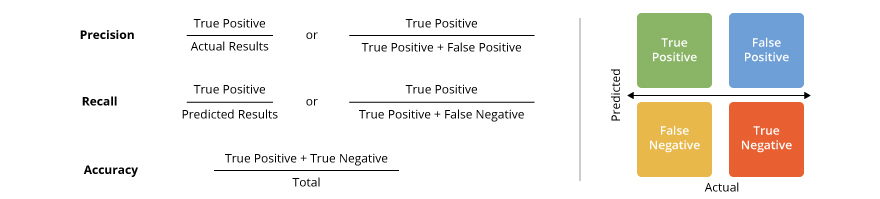Usually, data is distributed in different ways with a bias to the left or to the right or it can be all spilt across. However, there are chances that data is distributed around a central value without any bias to the left or right and reaches normal distribution in the form of a bell-shaped curve. A normal distribution is an arrangement of a data set in which most values cluster in the middle of the range and the rest taper off symmetrically toward either extreme.Figure: Normal distribution in a bell curve

The random variables are distributed in the form of a symmetrical bell-shaped curve.

Properties of Normal Distribution:

1. Unimodal - one mode
2. Symmetrical - left and right halves are mirror images
3. Bell-shaped - maximum height (mode) at the mean
4. Mean, Mode, and Median are all located in the centre
5. Asymptotic

K-means clustering is an unsupervised machine learning algorithm. It is the method of classification of data using a certain set of clusters as K clusters. It is mainly used to group data in order to find similarity in the data.It includes defining the K centres, one each in a cluster. The clusters are defined into K groups with K being predefined. The K points are selected at random as cluster centres. The objects are assigned to their nearest cluster centre. The objects within a cluster are as closely related to one another as possible and differ as much as possible to the objects in other clusters. K-means clustering works very well for large sets of data.

Random forest is a versatile machine learning method capable of performing both regression and classification tasks. It is also used for dimensionality reduction, treats missing values, outlier values. It is a type of ensemble learning method, where a group of weak models combine to form a powerful model.

In Random Forest, we grow multiple trees as opposed to a single tree. To classify a new object based on attributes, each tree gives a classification. The forest chooses the classification having the most votes (Overall the trees in the forest) and in case of regression, it takes the average of outputs by different trees.

SVM stands for support vector machine, it is a supervised machine learning algorithm which can be used for both Regression and Classification. If you have ‘n’ number of features in your training data set, SVM tries to plot it in n-dimensional space with the value of each feature being the value of a particular coordinate. SVM uses hyperplanes to separate out different classes based on the provided kernel function.The core algorithm for building a decision tree is called ID3. ID3 uses Entropy and Information Gain to construct a decision tree.

Entropy

A decision tree is built top-down from a root node and involve partitioning of data into homogenous subsets. ID3 uses entropy to check the homogeneity of a sample. If the sample is completely homogenous then entropy is zero and if the sample is an equally divided it has an entropy of one.Information Gain

The Information Gain is based on the decrease in entropy after a dataset is split on an attribute. Constructing a decision tree is all about finding attributes that return the highest information gain.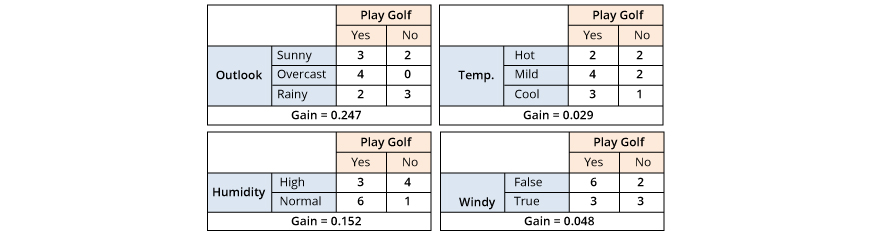The three types of analysis methodologies have single, double or multiple variables.

• Univariate analysis: This has only one variable and therefore there are no relationships, causes. The main aspect of the univariate analysis is to summarize the data and find the patterns within it to make actionable decisions.
• Bivariate analysis: This deals with the relationship between two sets of data. These sets of paired data come from related sources, or samples. Some of the tools used to analyze such data includes chi-squared tests and t-tests when the data have a correlation. The strength of the correlation between the two data sets will be tested in a Bivariate analysis.
• Multivariate analysis:  This is similar to bivariate analysis. It is a set of techniques used for the analysis of data sets that contain more than one variable, and the techniques are especially valuable when working with correlated variables.

When you are performing a hypothesis test in statistics, a p-value helps you to determine the strength of your results. P-value is a number between 0 and 1. It will denote the strength of the results based on the values.

The null hypothesis is the claim which is on trial. If the p-value is low (≤ 0.05), it indicates strength against the null hypothesis which means you should reject the null hypothesis. On the other hand, high p-value (≥ 0.05) indicates strength for the null hypothesis and can accept the null hypothesis. If the p-value is 0.05, it indicates the hypothesis could go either way.

Data cleansing plays an important role in data analysis as data collection takes place from various sources. In this process, data records are detected and corrected, and is ensured that data is complete and accurate. Also, any irrelevant components of data are deleted or modified as per the needs. Data cleansing can also be deployed while performing data wrangling or batch processing. Lastly, after data is cleaned it is confirmed according to the data sets in the system. Data cleansing should be performed on a regular basis as inaccurate data levels can grow quickly, compromising database and decreasing business efficiency.In Machine learning and statistics, it is a common task to fit a model to a set of training data. Later this model can be used to predict or classify new data points. When the model fits the training data but does not have a good predicting performance and generalization power, we have an overfitting problem.

Regularization is the process of adding information or adding a tuning parameter to a model in order to induce smoothness and prevent overfitting. This is mostly done by adding a constant multiple to an existing weight vector. The constant can either be L1 (Lasso) or L2 (ridge).

In the example below, we see how three different models fit the same dataset.

We used different degrees of polynomials: 1 (linear), 2 (quadratic) and 3 (cubic).

Notice how the cubic polynomial "sticks" to the data but does not describe the underlying relationship of the data points.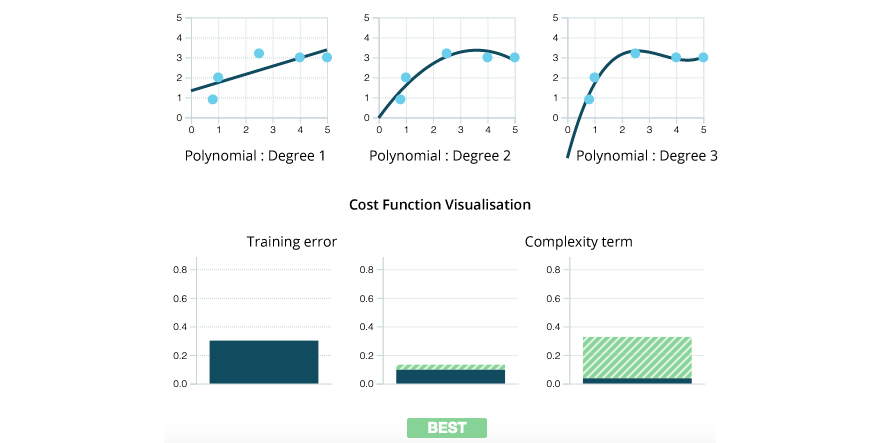Here, the regularization parameter λ = 2.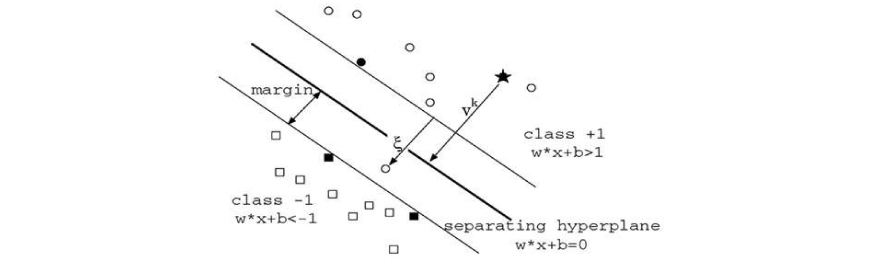In the above diagram, we can see that the thinner lines mark the distance from the classifier to the closest data points called the support vectors i.e the darkened data points. The distance between these two thin lines is called the margin. A Support Vector Machine (SVM) performs classification by finding the hyperplane that maximizes the margin between the two classes. The vectors (cases) that define the hyperplane are the support vectors.

There are four types of kernels in SVM.

1. Linear Kernel: It is used when the data is Linearly separable, that is, it can be separated using a single Line. It is one of the most common kernels to be used. It is mostly used when there are a Large number of Features in a particular Data Set. One of the examples where there are a lot of features, is Text Classification, as each alphabet is a new feature. So we mostly use Linear Kernel in Text Classification.
2. Polynomial kernel: This kernel function is commonly used with support vector machines (SVMs) and other kernelized models, that represents the similarity of vectors (training samples) in a feature space over polynomials of the original variables, allowing learning of non-linear models.
3. Radial basis kernel: It is also called the RBF kernel, or Gaussian kernel, which is in the form of a radial basis function (more specifically a Gaussian function).
4. Sigmoid kernel: This can be used as the proxy for neural networks.

There is no fixed answer to these questions, it depends largely on the dataset. However, there has to be a balance/equilibrium while you allocate data for training, validation and test sets.

If the training set selected is too small, the actual model parameters might have high variance. Again if the test set is too small there are possibilities of unreliable estimation of model performance. A general rule to follow would be to use an 80:20 train/test split, after which the training set can further be split into validation sets.

The answer totally depends on the domain for which we are trying to solve the question.

If we consider medical testing, false negatives might give a falsely reassuring message to patients and physicians that the disease is absent. This might sometimes lead to inappropriate or inadequate treatment of both the patients and disease. So it is desired to have more false positives.

Again, if we consider spam filtering, a false positive occurs when spam filtering techniques wrongly classify a valid email as spam. Most anti-spam tactics block or filter a good number of unwanted emails, doing so without creating significant false-positive results is a much more demanding task. So, we prefer too many false negatives over many false positives.

One of the most common tasks in statistics and machine learning is to fit a model to a set of training data in order to predict according to general untrained data.

Overfitting takes place when a model is excessively complex i.e if it has too many parameters compared to the number of observations. Overfitting leads to poor predictive performance as it overreacts to even minor fluctuations in the training data.

On the other hand, underfitting takes place when a model cannot capture the underlying trend of the data. For example, underfitting would occur while fitting a linear model to non-linear data resulting in poor predictive performance.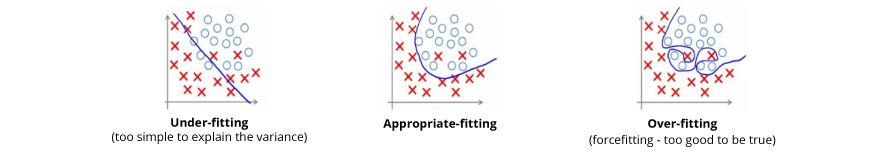Low bias occurs when the value predicted by the model is near to actual values. This happens when the model becomes flexible and mimics the training data distribution. In such cases, bagging algorithm (such as a random forest) can be used to handle low bias and high variance problem. This algorithm splits the data set into subsets which are made with repeated randomized sampling and the samples are used to develop a set of models using a single learning algorithm. Further, the predictions made by the model are combined using averaging (regression) or voting (classification).

In order to identify groups, we use classification technique, however, the regression technique is used to predict a response. Although both the techniques are related to prediction, where classification is used to predict the belonging to a class whereas regression predicts the value from a continuous set.

Classification technique is used over regression when the results of the model need to return the belongingness of data points in a dataset to specific explicit categories. For example, suppose you want to find out whether a name is male or female you will use classification technique, however, to find how correlated they are with male and female names you will have to go for regression technique.

A confusion matrix is a 2X2 table which contains 4 outputs which are provided by the binary classifier. Some of the measures, such as accuracy, error-rate, sensitivity, specificity, precision and recall, these are derived from it.The data set which is used for performance evaluation is called as the test data set. It contains the correct labels and predicted labels.The predicted labels will exactly be the same if the performance of a binary classifier is perfect.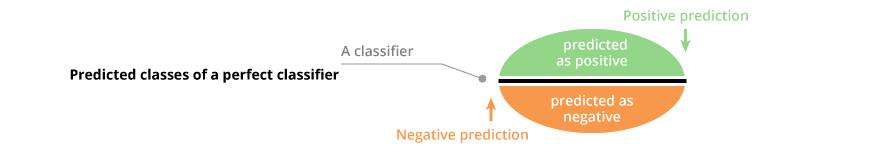The predicted labels usually match with part of the observed labels in real-world scenarios.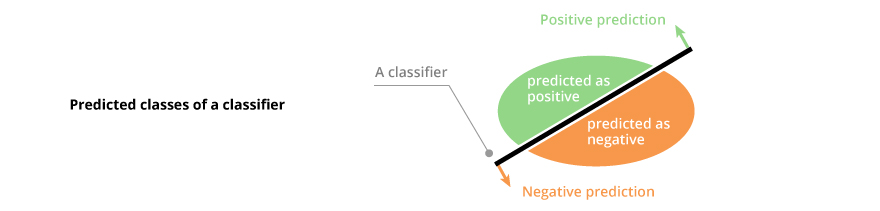A binary classifier predicts all data instances of a test dataset as either positive or negative. This produces four outcomes-

1. True positive(TP) — Correct positive prediction
2. False positive(FP) — Incorrect positive prediction
3. True negative(TN) — Correct negative prediction
4. False negative(FN) — Incorrect negative predictionBasic measures derived from the confusion matrix

1. Error Rate = (FP+FN)/(P+N)
2. Accuracy = (TP+TN)/(P+N)
3. Sensitivity(Recall or True positive rate) = TP/P
4. Specificity(True negative rate) = TN/N
5. Precision(Positive predicted value) = TP/(TP+FP)
6. F-Score(Harmonic mean of precision and recall) = (1+b)(PREC.REC)/(b²PREC+REC) where b is commonly 0.5, 1, 2.

Some of the methods to validate a model are:

• If the predicted values by the model are far outside the response variable range, it will indicate poor estimation or model accuracy
• Examine the parameters if the values seem to be reasonable. Also, check if:
• opposite signs of expectations, or
• unusually large or small values, or
• observed inconsistency when the model is fed new data

In such cases, it will indicate poor estimation or multicollinearity.

• Feed new data and use the model for prediction. Also, use the coefficient of determination (R squared) as a model validity measure
• Split the data to form a separate dataset for estimating model parameters and another for validating predictions

Building a decision tree mainly involves 4 steps:

1. You need to consider the training dataset, which should have some feature variables and classification or regression output.
2. Now you will have to determine the “best feature” in the dataset to split the data. The best feature and the specific split is commonly chosen using a greedy algorithm to minimize a cost function.
3. Once the feature is selected, you will have to split the data into subsets that contain the possible values for this best feature. This splitting basically defines a node on the tree i.e each node is a splitting point based on a certain feature from our data.
4. Recursively generate new tree nodes by using the subset of data created from step C. We keep splitting until we reach a point where we have optimised, by some measure, maximum accuracy while minimising the number of splits/nodes.

The algorithm is ‘naive’ because it makes assumptions that may or may not turn out to be correct. Naïve Bayes algorithm is considered Naïve because it makes the assumption that features of measurement are independent of each other. It is said to be naive as it is (almost) never true. The assumptions this algorithm make are virtually impossible to find in real-life data. The conditional probability is actually the pure product of individual probabilities of components, which means that the algorithm assumes the presence or absence of a specific feature of a class is not related to the presence or absence of any other feature (absolute independence of features), given the class variable.
For example, if you consider a banana which is yellow, long and about 5 inches in length, and if these features depend on each other or are based on the existence of other features, a naïve Bayes classifier will assume all these properties to contribute independently to the probability that this fruit is a banana.

## Description

Data science is one of the most promising and in-demand career paths for skilled professionals. Data professionals need the skills of analyzing large amounts of data, data mining, and programming skills.

As per the Glassdoor Data Scientist has become one of the best jobs in America in 2018. The demand for data science professionals across various industries is being affected by a shortage of qualified candidates available to fill the open positions. Let’s understand the demand for Data science professionals. With 4,500 open positions listed on Glassdoor, data science professionals are rewarded high. Data science professionals with the right skills sets, experience have got a very good opportunity to reserve their job in some of the most forward-thinking companies in the world.

According to Glassdoor the average salary of a Data Scientist is \$117,345. Experience plays a vital role when it comes to the salary of a Data Scientist. 2016 Data Science Salary Survey states that experience is one of the most important factors in a Data Scientist’s salary.

If you’re looking for Data Science Python interview questions for experienced and freshers, then you are at the right place. There are a lot of opportunities in many reputed companies across the globe. Good hands-on knowledge concepts will put you forward in the interview. You can find job opportunities everywhere. Our Data Science technical interview questions are exclusively designed for supporting employees in clearing interviews. We have tried to cover almost all the main topics related to Data Scientists.

Here, we have characterized the questions based on the level of expertise you’re looking for. Preparing for your interview with these Data Science coding interview questions will give you an edge over other interviewees and will help you crack the Data Scientists interview.

Stay focused on the essential common Data Science interview questions and prepare well to get acquainted with the types of questions that you may come across in your interview on Data Scientists. You can also take up the Data Science training to enrich your knowledge.

Hope these Data science python interview questions will help you to crack the interview. All the best!